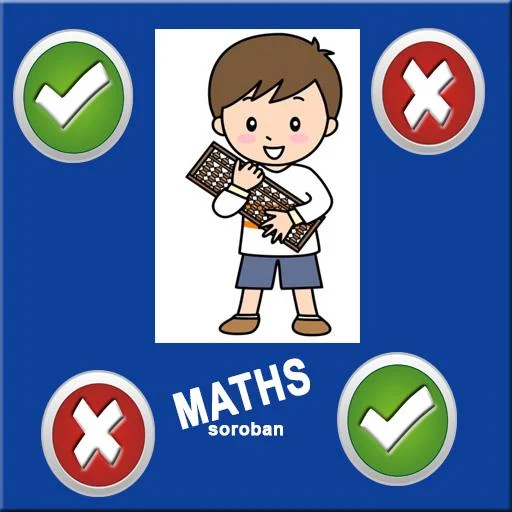-->

# Androide game: math soroban gameMath soroban game: Crack your brain,  let's test our maths calculation speed.

Can you do fast maths calculation? let's test our maths calculation speed. "Math soroban game" Mathematical Game will help you to improve your maths brain calculation as interactive and fun way.

Math soroban game : Crack your brain with this Android quiz type maths game. User just answers simple maths problems on TRUE OR FALSE. It’s most popular android app

Can you do fast maths calculations? let’s test your maths calculation speed. “Math soroban game” Mathematical Game will help you to improve your maths skill in interactive and fun way.@مرسلة بواسطة
كاتب ومحرر اخبار اعمل في موقع مدونة سوروبان العرب للحساب الذهني و الذكاء و تعليم الطفل .